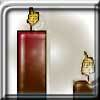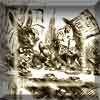#### You may also like### Burning Down

One night two candles were lit. Can you work out how long each candle was originally?### A Problem of Time

Consider a watch face which has identical hands and identical marks for the hours. It is opposite to a mirror. When is the time as read direct and in the mirror exactly the same between 6 and 7?### Fair Shares?

A mother wants to share a sum of money by giving each of her children in turn a lump sum plus a fraction of the remainder. How can she do this in order to share the money out equally?

# Fractions of Fractions

##### Age 14 to 16 ShortChallenge Level

Two thirds of five sixths of a number $X$ is the same as three quarters of four fifths of a number $Y$.

What is the value of $\frac{X}{Y}$ as a fraction in its lowest terms?

If you liked this problem, here is an NRICH task which challenges you to use similar mathematical ideas.

This problem is taken from the UKMT Mathematical Challenges.
You can find more short problems, arranged by curriculum topic, in our short problems collection.